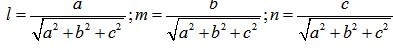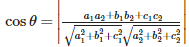# Three Dimensional Geometry - Online Test

Q1. Direction cosines of a line are
Explaination / Solution:

a line when intersect another line or axis two kind of angle form ,as an assumption we take positive side of axis, to define the direction of line we take angle made from all three axis.and we take cos not other trigonometric function like sin,tan because we can define Direction cosines of a line are coefficient of i,j,k of a unit vector along that line.

Q2. If l, m, n are the direction cosines of a line, then
Explaination / Solution:

we know that direction cosines is coefficient of i,j,k of unit vector along that line ,i.e those coefficient are l,m,n .the length of the line r is such that  and magnitute of unit vector r is 1 and . on squaring both side we get
Q3.

is a vector joining two points P(x1, y1, z1) and Q(x2, y2, z2).If ,Direction cosines of are

Explaination / Solution:

since we know Direction cosines of a line are coefficient of i,j,k of a unit vector along that line,first find a vector   then to convert  it unit vector divide by its magnitute || the coefficient of this unit vector will be
Q4. If l, m and n are the direction cosines of a line, Direction ratios of the line are the numbers which are
Explaination / Solution:

If l, m and n are the direction cosines of a line, Direction ratios of the line are the numbers which are Proportional to the direction cosines of the line.

Q5. If l, m, n are the direction cosines and a, b, c are the direction ratios of a line then
Explaination / Solution:

using direction ratio a,b,c we got a vector  along  the line ,for direction cosine find  unit vector thenwhere l,m,n represent direction cosine

Q6. Skew lines are lines in different planes which are
Explaination / Solution:

By definition : The Skew lines are lines in different planes which are neither parallel nor intersecting .

Q7. Angle between skew lines is
Explaination / Solution:

Angle between skew lines is the angle between two intersecting lines drawn from any point parallel to each of the skew lines .

Q8. If  and  are the direction cosines of two lines; and  is the acute angle between the two lines; then
Explaination / Solution:

If  and  are the direction cosines of two lines; and  is the acute angle between the two lines; then the cosine of the angle between these two lines is given by :  .

Q9. If  and  are the direction ratios of two lines and  is the acute angle between the two lines; then
Explaination / Solution:

If  and  are the direction ratios of two lines and  is the acute angle between the two lines; then , the cosine of the angle between these two lines is given by :Q10. Vector equation of a line that passes through the given point whose position vector is     and parallel to a given vector  is
Explaination / Solution:

Vector equation of a line that passes through the given point whose position vector is    and parallel to a given vector     is given by :